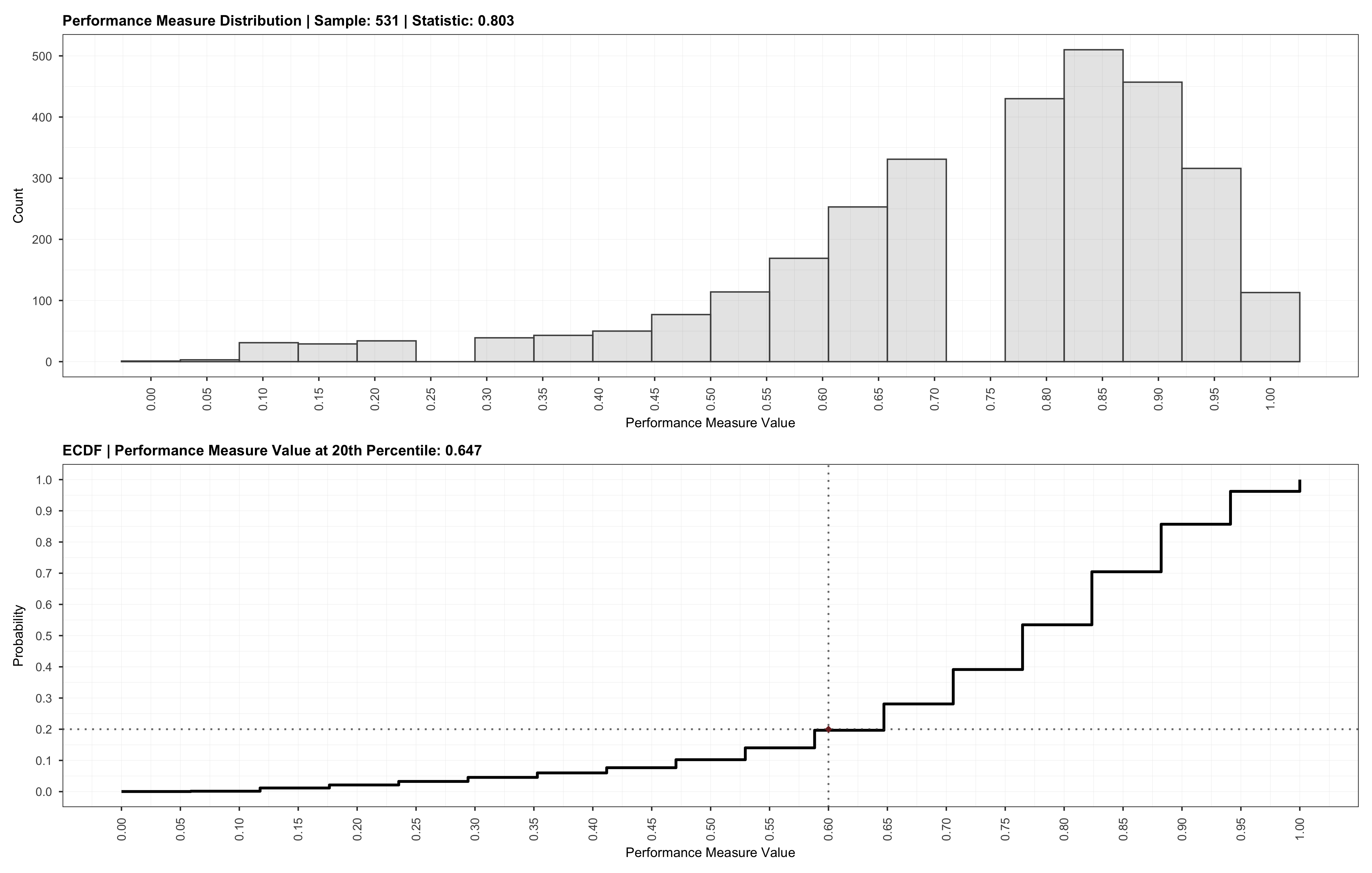# # Plot Validation Results

## # Description

The `plot.Validation` `S3` methodopen in new window plots the results of a sample size analysis validation.

## # Usage

``````plot(
x,
save = FALSE,
path = NULL,
width = 14,
height = 10,
bins = 20,
...
)
``````

## # Arguments

NameDescription
`x`An object instance of class `Validation` produced by the function `validate`.
`save`A logical value indicating whether the plot should be saved to a file on disk.
`path`A character string representing the path (i.e., including the filename and extension) where the plot should be saved on disk. If `NULL`, the plot will be saved in the current working directory with a filename generated based on the current system time and a `.pdf` extension. See `ggplot2::ggsave`open in new window for supported file types.
`width`A single numerical value representing the desired plot width. The default unit is inches (i.e., set by `ggplot2::ggsave`open in new window), unless overridden by providing the `units` argument via `...`.
`height`A single numerical value representing the desired plot height. The default unit is inches (i.e., set by `ggplot2::ggsave`open in new window), unless overridden by providing the `units` argument via `...`.
`bins`A single positive integer passed to `ggplot2::geom_histogram`open in new window representing the number of bins to use for the histogram plot. The default value is `20`.
`...`Optional arguments to be passed to `ggplot2::ggsave`open in new window.

## # Return

An `ggplot2::ggplot`open in new window object containing the plot for the validation procedure. The plot object returned can be further modified and also contains the `patchwork`open in new window class applied. An example of a validation plot is shown below.

Sample Size Analysis Validation`S3` method `summary` and function `validate`.